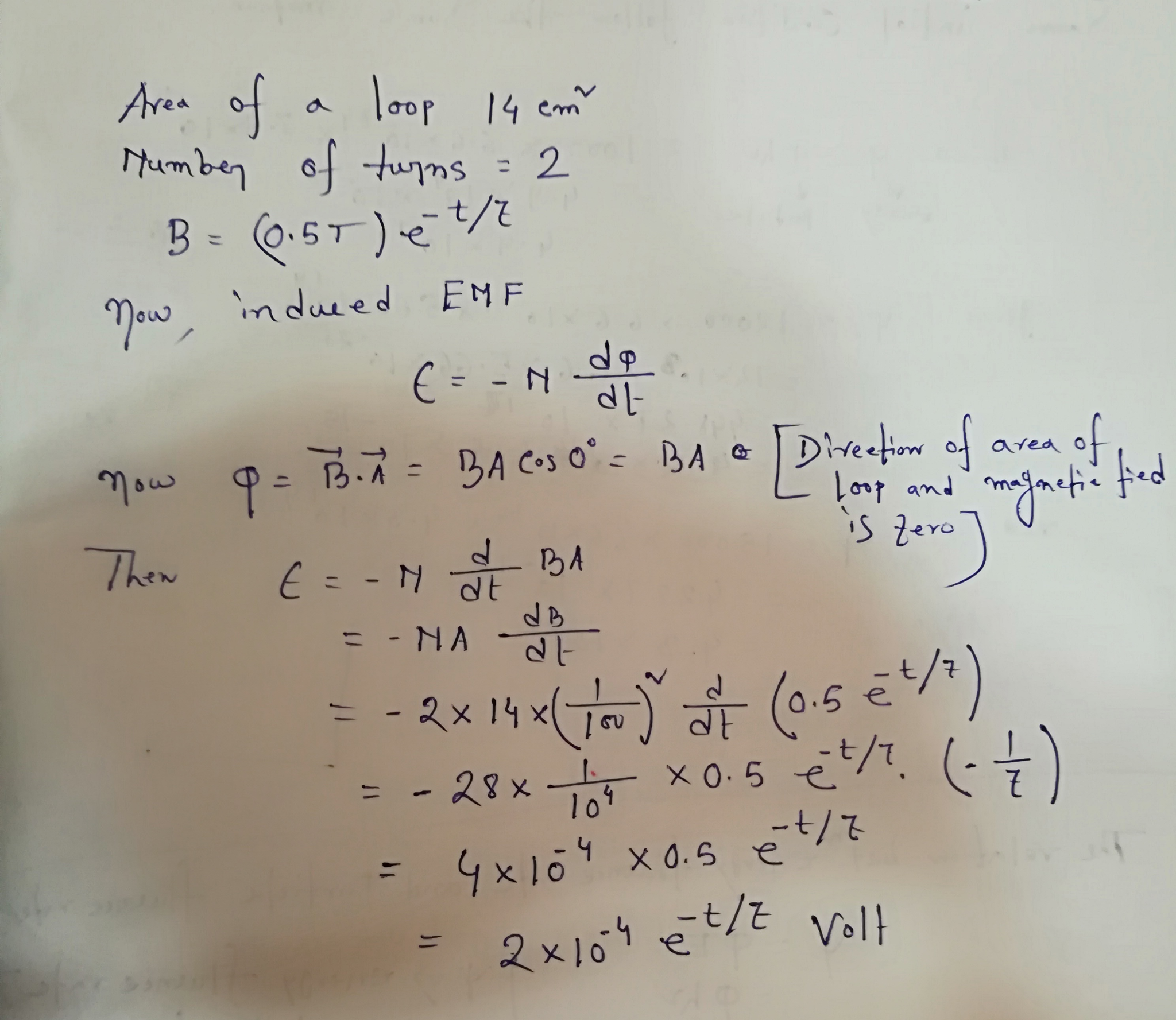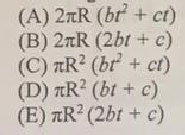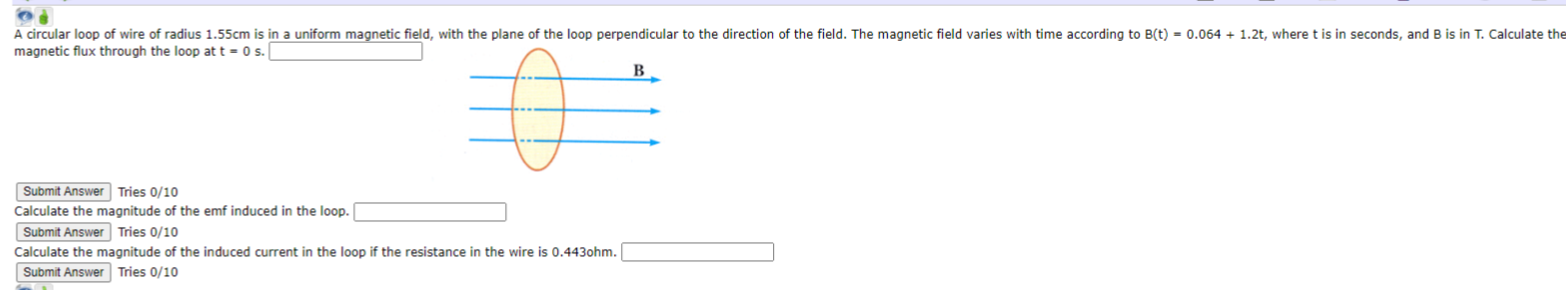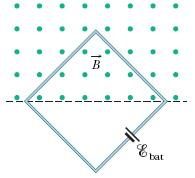Question

A plane loop of wire of area 14cm2 with two turns is perpendicular to a magnetic field whose magnitude decays in time according to B= (0.5 T)e-t/7. What is the induced EMF as a function of time?#### Earn Coins

Coins can be redeemed for fabulous gifts.

Similar Homework Help Questions
• ### A magnetic field of magnitude 1.42 T is perpendicular to the plane of a circular wire loop. As th...

A magnetic field of magnitude 1.42 T is perpendicular to the plane of a circular wire loop. As the magnetic field decreases to zero in a time of 0.0314 s, the average induced emf is 0.284 V. What is the radius of the loop?

• ### a circular loop of wire radius r is perpendicular to a magnetic field whose magnitude as...a circular loop of wire radius r is perpendicular to a magnetic field whose magnitude as a function of time t is given by the equations B=bt^2 + ct, where b and c are positive, nonzero constants. What is the magnitude of the emf induced in the loop as a function of time t?

• ### A horizontal square wire loop of side d lies in a plane perpendicular to a uniform...

A horizontal square wire loop of side d lies in a plane perpendicular to a uniform magnetic field B pointing from above into the plane of the loop. The magnitude of magnetic eld ~ B is: B = 0.80 T. If in ∆t = 4.0 ms the wire is reshaped from a square to a rectangle of dimensions: L = 10.0 cm; W = 6.0 cm, but remains in the same plane. a - What is the side d of...

• ### A magnetic B field of strength 16 T is perpendicular to a conducting loop of wire...

A magnetic B field of strength 16 T is perpendicular to a conducting loop of wire measuring 0.6 square meters with 40 turns. If the B field is linearly reduced to zero in 6 s, then what is the magnitude of the induced emf voltage?

• ### os A circular loop of wire of radius 1.55cm is in a uniform magnetic field, with...os A circular loop of wire of radius 1.55cm is in a uniform magnetic field, with the plane of the loop perpendicular to the direction of the field. The magnetic field varies with time according to B(t) = 0.064 + 1.2t, where t is in seconds, and B is in T. Calculate the magnetic flux through the loop at t0 s. B Submit Answer Tries 0/10 Calculate the magnitude of the emf induced in the loop. Submit Answer Tries 0/10...

• ### A magnetic B field of strength 4 T is perpendicular to a conducting loop of wire...

A magnetic B field of strength 4 T is perpendicular to a conducting loop of wire measuring 0.4 square meters with 50 turns. If the B field is linearly reduced to zero in 2 s, then what is the magnitude of the induced emf voltage? (in units of V)

• ### (a) A square loop of wire with sides of length 40cm is in a uniform magnetic...

(a) A square loop of wire with sides of length 40cm is in a uniform magnetic field perpendicular to its area. if the field's strength is initially 100mT and it decays to zero in 0.010 s, what is the magnitude of the average emf induced into the loop? (b) What is the average emf if the sides of the loop were only 20cm?

• ### A square wire loop with 2.0 m sides is perpendicular to a uniform magnetic field, with...A square wire loop with 2.0 m sides is perpendicular to a uniform magnetic field, with half the area of the loop in the field as shown in Figure 30-41. The loop contains an ideal battery with emf = 16 V. If the magnitude of the field varies with time according to B = 0.050 - 0.88 t, with B in teslas and t in seconds, what are (a) the magnitude and (b) the direction of the net emf in...

• ### The loop is in a magnetic field 0.26 T whose direction is perpendicular to the plane of the loop

The loop is in a magnetic field 0.26 T whose direction is perpendicular to the plane of the loop. At t = 0, the loop has area A= 0.285 m2 .Suppose the radius of the elastic loop increases at a constant rate, dr/dt =3.75 cm/s. Part A Determine the emf induced in the loop at t=0 and at t=1.00 s. Part B Express your answer using two significant figures.

• ### A uniform magnetic field is at right angles to the plane of a wire loop. The...

A uniform magnetic field is at right angles to the plane of a wire loop. The field decreases by 0.16 Tin 3.0×10−3 s and the magnitude of the average emf induced in the loop is 79 V . A) What is the area of the loop? (in m^2) B) What would be the value of the average induced emf if the field change was the same but took twice as long to decrease? (in Volts)